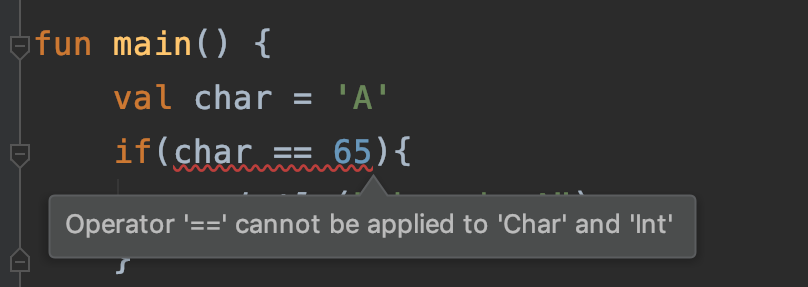Owen LeeKotlin 修炼手册（2）基本数据类型

#### 数值类型

Kotlin 中的数值类型有：

Byte 字节 8 byte(Byte)
Short 短整型 16 short（Short）
Int 整型 32 int(Integer)
Long 长整型 64 long(Long)
Float 浮点型 32 float(Float)
Double 双精度浮点型 64 double(Double)

1. Long 类型要以 L 结尾
2. Double 类型写法：3.14，1.5e10，1.5E10
3. Float 类型要加 fF 后缀：3.14f
4. 十进制：123
5. 二进制以 0b 开头：0b0001
6. 八进制：不支持
7. 十六进制以 0x开头：0xFFF
8. 使用下划线使数字常亮更易读：1_000_000
val a1: Byte = 1
val a2: Short = 1
val a3: Int = 1
val a4: Long = 1L
val a5: Float = 3.14f
val a6: Double = 3.14

//二进制
val a7 = 0b0001
//十六进制
val a8 = 0xFFF
//使用下划线
val oneMillion = 1_000_000
val phoneNumber = 137_6730_5920L
val argb  = 0xFF_00_EF_35
val bytes = 0b11000011_00100100

===：用来比较两个在内存地址上是否相等
==：用来比较两个数值是否相等

val a1 = 2000
val a2 = 2000

fun main() {
println("a1 == a2:${a1 == a2}") println("a1 === a2:${a1 === a2}")
}
//运行结果
a1 == a2:true
a1 === a2:true

val a1 = 2000
val a2 :Int? = a1
val a3 :Int? = a1
fun main() {
println("a2 == a3:${a2 == a3}") println("a2 === a3:${a2 === a3}")
}
//运行结果
a2 == a3:true
a2 === a3:false

val a1 = 2000
val a2 :Int = a1
val a3 :Int = a1
fun main() {
println("a2 == a3:${a2 == a3}") println("a2 === a3:${a2 === a3}")
}
//运行结果
a2 == a3:true
a2 === a3:true

val a1 = 2
val a2 :Int? = a1
val a3 :Int? = a1
fun main() {
println("a2 == a3:${a2 == a3}") println("a2 === a3:${a2 === a3}")
}
//运行结果
a2 == a3:true
a2 === a3:true

Kotlin基本类型自动装箱的一点问题

Java 笔试面试（4）基本数据类型与运算

toByte() - 转换成字节型
toShort() - 转换成短整型
toInt() - 转换成整型
toLong() - 转换成长整型
toFloat() - 转换成浮点型
toDouble() - 转换成双精度浮点型
toChar() - 转换成字符类型
toString() - 转换成字符串类型

val a = 1L + 3
// Long + Int => Long

shl(bits) – 左移位 (等价于Java里的 <<)
shr(bits) – 右移位 (等价于Java里的 >>)
ushr(bits) – 无符号右移位 (等价于Java里的 >>>)
and(bits) – 与
or(bits) – 或
xor(bits) – 异或
inv() – 反向

#### 布尔类型

Kotlin 中逻辑操作符和 Java 中是一样的。

&& ：短路逻辑与
|| ：短路逻辑或
!：取非

#### 字符类型

Kotlin字符类型用 Char （大写），要注意的是：fun main() {
val char = 'B'
if(char.toInt() == 65){
println("char is A")
}
}

#### 字符串类型

Kotlin 中 String 表示字符串，和 Java 一样是不可变的。在 Kotlin 中，可以通过数组下标的方式（string[index]）来取字符，也可以使用 in 关键字来遍历整个字符串。

fun main() {
val str: String = "hello"
println("内容：$str") println("第一个字符是：${str}")
println("第二个字符是：${str.get(1)}") for (s in str){ println(s) } } //运行结果 内容：hello 第一个字符是：h 第二个字符是：e h e l l o Kotlin 支持多行字符串，用 """ """ 表示。 fun main() { val str: String = """ one two three """ println("内容：$str")
}
//运行结果

one
two
three

#### 数组类型

1. arrayOf() 方法，参数是可变长的泛型对象
val a = arrayOf(1, 2, 3)
val b = arrayOf("one", "two", "three")
1. arrayOfNulls，可以创建一个指定类型的初始值全为空的数组，参数是数组长度。
fun main() {
val arr = arrayOfNulls<String>(3)
for(a in arr){
print("$a ") } arr = "one" arr = "two" arr = "three" println() for(a in arr){ print("$a ")
}
}
//运行结果
null null null
one two three 
1. 工厂函数：Array(size, {i -> (包含 i 的表达式)})

fun main() {
val arr = Array(3, {i -> i * 2 })
for(a in arr){
print("\$a ")
}
}
//运行结果
0 2 4 

Kotlin 中还有 ByteArray、ShortArray、IntArray 等类用来表示各个类型的数组，这些类省去了装箱操作，效率更高，使用方式和 Array 一样。

ByteArray - 表示字节型数组
ShortArray - 表示短整型数组
IntArray - 表示整型数组
LongArray - 表示长整型数组
BooleanArray - 表示布尔型数组
CharArray - 表示字符型数组
FloatArray - 表示浮点型数组
DoubleArray - 表示双精度浮点型数组